# Denoising Electrocardiogram Signal from Electromyogram Noise Using Adaptive Filter Combination

Denoising Electrocardiogram Signal from Electromyogram Noise Using Adaptive Filter Combination
Laboratoire de Recherche Modélisation, Simulation et Optimisation des Systèmes Complexes Réels, Université ZIANE Achour de Djelfa, Ain Chih, Djelfa 17000, Algérie

Corresponding Author Email:
an.khiter@gmail.com
Page:
67-74
|
DOI:
https://doi.org/10.18280/ria.340109
18 October 2019
|
Accepted:
20 December 2019
|
Published:
29 February 2020
| Citation

OPEN ACCESS

Abstract:

The electrocardiogram (ECG) signal is the most important diagnostic test of heart disease detection; it is characterized by low frequency and weak amplitude which makes it susceptible to different kinds of noises such as high/ low-frequency noises. Thus, the diagnostic quality is reduced. This paper introduces a new filter that take the output of wavelet wiener based filter to design a normalized Least Mean Square (NLMS) based filter in order to reduce the broadband mypotentials (EMG) noise in ECG signal. Testing is performed by taking ECG signal from standard MIT/BIH arrhythmia database sampled at 360 Hz-per second and the model of the EMG noise is generated by shaping white Gaussian noise before we add it to a clean ECG signal to get the noisy signal. The proposed method gives best noise reduction and preserves the ECG waves shape. In addition, the combination shows better results through increasing SNR and reducing Mean Square Error (MSE) compared to other existing techniques.

Keywords:

ECG signal, EMG noise, wavelet transform (WT), wiener filtering, normalized least mean square algorithm (NLMS)

1. Introduction

The Electrocardiogram (ECG) is one important signal among bioelectrical signals; it reflects the electrical activity of the human heart and illustrates the sequences of atrial depolarization wave (P wave), a ventricular depolarization wave (QRS complex) and a ventricular repolarization wave (T wave) as presented in Figure 1 . Since ECG is a low amplitude signal and contains low-frequency waves, it is very sensitive to different internal or external noises such as high/ low-frequency noises, one of the important high frequency noises being the electromyographic noise (EMG noise), which is caused by electrical activity of skeletal muscles during periods of contraction. Its frequency components are considerably overlapping the ECG spectrum. EMG noise is the most difficult type of broadband myopotentials noise to remove because it causes widening of the QRS complex, cropping of peaks in QRS complexes and completely mask PQ and ST intervals, the P- and T-waves [1, 2]. There are many methods that have been implemented to eliminate the noise from noisy signal such as nonlinear filter banks , adaptive filtering [4, 5], principal component analysis (PCA) and independent component analysis (ICA) , Genetic Particle Filtering , wavelet transform [8, 9], Empirical mode decomposition (EMD) and non-local mean (NLM) technique . The filters possess disadvantages that they remove important frequency components in the vicinity of cut-off frequency, adaptive filters have the ability to adjust their parameters automatically and don’t need a prior knowledge of signal or noise description. Empirical mode decomposition technique is not perfect method in processing non-stationary signal such as ECG signal. Wavelet-based theory is different from Fourier analysis and spectral theory since it is based on a local frequency representation where the wavelet denoising technique does not require a reference nor needs multi-channel signals.

Nikolov and Gotchev  proposed an algorithm for ECG signal denoising in the wavelet domain. The coefficients were shrunk by applying a time and frequency dependent threshold. The amplitudes of the QRS complex are preserved and the noise is reduced. However, artifacts could arise in the transient PQ and ST. Nikolov and Gotchev  apply wavelet domain wiener filtering where the last problem was avoided. A denoising using combination between wavelet Shrinkage with Wiener filtering in translation-invariant wavelet domain was proposed which led to a reduction in the oscillation around QRS complex . Li and Lin  proposed an optimal denoising algorithm for ECG signal using stationary wavelet transform where they conclude that by using Sym4, decompose the signal at level 5, and Hard shrinkage function with EBayes threshold can get the better de-noising effect before automatic evaluation or clinic analysis. Chmelka and Kozumplik  proposed the Wiener filtering in shift-invariant wavelet domain with pilot estimation of the signal. The four-levels with hybrid thresholding were used for pilot estimation. Smital et al.  proposed an Adaptive Wavelet Wiener Filtering of ECG Signals, where a block of noise estimate was added, the approach provides better results than the classic wavelet Wiener filter. El B’charri et al.  proposed dual tree wavelet transform (DT-WT) to denoise and enhance ECG signal, the results have shown that the modified unified threshold with wavelet hyperbolic threshold denoising method is better in realistic and colored noises than the ordinary dual tree wavelet transform. Roopkanwal and Kaur  proposed a novel modified DWT-FFT-FXLMS algorithm for ECG waveform denoising, the method achieved results with better performance in terms of the number of performance measures for ECG signal altered by diverse noises such as EMG noises. Kumar et al.  proposed empirical mode decomposition (EMD) with non-local mean (NLM) technique, EMD framework the was used for reduction of the noise from the ECG signal, then the output of the EMD passes through NLM framework for preservation of the edges, The method has showed lesser mean of percent root mean square difference (PRD), mean square error (MSE), and better mean SNR improvement.

In this paper we introduce a new filter consists of two stages, first the noisy ECG signal passes through wavelet wiener filter where 59 filter banks and five thresholding techniques were tested using Log Scale Modified Universal (LSMU) threshold, then the output of the first filter is taken to design an adaptive filter which based on NLMS algorithm where the step size becomes adaptive. The main objective of this work is not only to clean the noisy ECG signal but also to get the signal with all its waves and characteristics without distortion; with lesser Mean Square Error (MSE), and better SNR improvement. The organization of the paper is as follows: The proposed new combination based on adaptive wavelet wiener filter with Normalized Least Mean Square (NLMS) algorithm using Log Scale Modified Universal (LSMU) threshold is explained in Section 2. The results and discussion are presented in Sections 3 and 4, respectively. Final section presents the conclusion.

## 1.png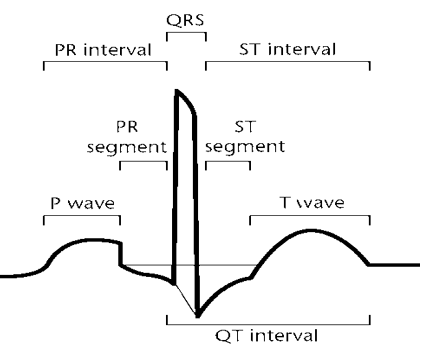Figure 1. Normal ECG signal

2. Materials and Methods

2.1 Stationary wavelet transforms (SWT)

The wavelet transform is an effective tool in the ECG signal denoising and it is the best choice then classical filtering as it exploits frequency and time content which does not happen in typical filter design. According to the literature in WT de-noising domain also from the experience of authors dealing with signal processed [18, 19], it has been tested that using Cyclic Shift Tree, TI (Shifted Invariant) or SWT can improve SNR and reduce the Pseudo-Gibbs phenomenon. The SWT is similar to the classical discrete WT (DWT), except the signal is never sub-sampled, and the filters are up-sampled at each level of decomposition. Thus, interpolation wrongs that might occur during the reconstruction step can be avoided [15, 9]. The SWT signal denoising consists of three procedures:

1) Signal decomposition; where the noisy ECG signal is transformed to wavelet domain to find SWT coefficients up to appropriate level j (level of decomposition which denotes the jth frequency band); the procedure results in signal decomposition: lower bands with more components of QRS complex (approximate coefficients) and highest bands with EMG noise along with some additive components of QRS complex (detail coefficients)

2) SWT coefficients thresholding method; The algorithm was proposed by Donoho and Johnstone  applied on detail coefficients, it is varied based on types and rules to obtain the estimated wavelet coefficients that are calculated from 1 to j, this step needs to choose and estimate the optimal thresholding algorithms and the optimal threshold value.

3) Reconstruction of the denoised signal by applying the Inverse Wavelet Transformation (IWT) to coefficients.

The corrupted signal denoted by x(n) is an additive mixture of noise-free signal s(n) and noise w(n) as illustrated in Eq. (1) as follows:

x(n)=s(n)+w(n)    (1)

n represents the discrete time (n=0,1…., N-1) and N is the length in samples of the time-domain signal.

In Eq. (2) the noisy signal x(n) is transformed into wavelet domain to obtain wavelet coefficients as follows:

$\mathrm{y}_{j}(\mathrm{n})=\mathrm{u}_{j}(\mathrm{n})+\mathrm{v}_{j}(\mathrm{n})$    (2)

where, $u_j$: are coefficients of noise free signal and $v_j$  are the coefficients of the noise.

The choice of decomposition-reconstruction filter banks, the level of decomposition, the threshold value and the strategy of thresholding method are important and affect the quality of noise suppression. And according to the selected values, the de-noised ECG signal could either retain some interference or have some distortion and discontinuities.

In this paper 59 filter banks were tested, Haar, DB, sym, coif, bior, rbio, dmey and fk with different vanishing moments (which are present in the MATLAB Wavelet Toolbox);

There are many methods for estimating the optimal threshold values . For our combination Log Scale Modified Universal Method (LSMU) was used , this method has an advantage over others (Length Modified Universal Method, Log Variable Modified Universal Method, Scale Length Modified Universal Method, Minimax threshold ….)  that it takes different thresholds at different scales. LSMU threshold is progressively reduced, and it is accordant with the distribution characteristics of the noise in different scales of the wavelet transform to ensure the efficiency of the denoising technique; it is given by Eq. (3) as follows:

$\mathrm{TSH}_{\mathrm{LSMU}}=\frac{\sqrt{2 \log (\mathrm{N})}}{\log (\mathrm{i}+1)} \cdot \sigma_{\mathrm{v j}}$    (3)

The parameter $\sigma_{\mathrm{v} j}$ is the standard deviation of noise on j-th decomposition level, and log is a natural logarithm. $\sigma_{\mathrm{v} j}$ can be estimated using the median parameter  which can be calculated as given by Eq. (4) as follows:

$\sigma_{v j}=\frac{\operatorname{median}\left(\left|y_{j}\right|\right)}{0.6745}$    (4)

where, $\frac{1}{0.6745}$ is a constant scale factor, it depends on distribution of the noise.

Five thresholding algorithms were tested in this paper: hard, soft, hyperbolic, nonnegative garrote and semisoft (firm) thresholding . In hard thresholding the coefficient absolute values below the threshold are set to zero, in soft thresholding first setting to zero the coefficient absolute values below the threshold and then shrinking the coefficients absolute values greater or equal the threshold towards zero. The hard routine creates discontinuities, while the soft routine is a continuous function but induces a biased estimation of large coefficients ; garrote is an intermediate between soft and hard. It acts like soft thresholding for small data values and approximates hard thresholding for large data values; firm routine requires two threshold values (threshold-high and threshold-low) acts as soft thresholding for values below threshold-low and the same as hard thresholding for values above threshold-high. For intermediate values, the thresholded value is in between that corresponding to hard or soft thresholding. Firm is a continuous function as soft thresholding, but is unbiased for large values as hard thresholding. Hyperbolic routine can be obtained by soft thresholding energies of the wavelet coefficients . Table 1 shows an example of some investigated parameters (decomposition level, thresholding methods and filter banks) for adaptation.

Table 1. Example of investigate parameters for adaptation

 Decomposition Level Thresholding Method Filter Bank WT1 Filter Bank WT2 3 Hyperbolic sym8 Db4 5 Semi-soft sym2 Sym2 6 Soft bior1.3 Sym8 4 Garrote sym8 bior1.1 4 Hard fk4 Dmey

2.2 Adaptive wavelet wiener filtering method (AWWF)

After the classic wavelet Wiener filter (WWF) approach proposed by Chmelka and Kozumplik  as illustrated in Figure 2, a block of noise estimate (NE) was added in the study , the approach based on the Wiener filtering theory applied in the wavelet domain .

## 2.pngFigure 2. Block diagram of the WWF method

WWF is based on two steps:

1)

• The input x(n) is decomposed to desired level j using wavelet filter bank 1(swt1 block) to obtain $y_{j}(n)$.
• Apply thresholding technique on detail coefficients.
• Reconstruct the denoised signal in ISWT1 block.
• Then using wavelet filter bank 2 (swt2 block) to decompose the reconstructed signal to obtain $\widehat{u}_{j}(n)$ the estimate of $u_{j}(n)$.

2)

• $y_{j}(n)$ and $\hat{u}_{j}(n)$ are used to design the Wiener Filter via the Wiener correction factor as given by Eq. (5):

$\hat{g}_{j}(n)=\frac{\hat{u}_{j}^{2}(n)}{\hat{u}_{j}^{2}(n)+\sigma_{v j}^{2}(n)}$(5)

To obtain the modified coefficients $\lambda_{y_{j}(n)}$ given by Eq. (6) as follows:

$\lambda_{y_{j}(n)}=y_{j}(n) \cdot \hat{g}_{j}(n)$    (6)

• The output y(n) signal is obtained by taking inverse stationary wavelet transform 2 of modified coefficients $\lambda_{y_{j}(n)}$.

In AWWF (Figure 3), The NE block needs two inputs:

• The noisy signal x(n).
• And the estimate of the noise-free signal y(n) obtained by the WWF method. The difference between these two signals gives an estimate of the input noise and thus SNR can be calculated.

The parameters in WWF block are set up using the estimated SNRest value. Then the output signal z(n) is obtained by taking inverse stationary wavelet transform.

## 3.png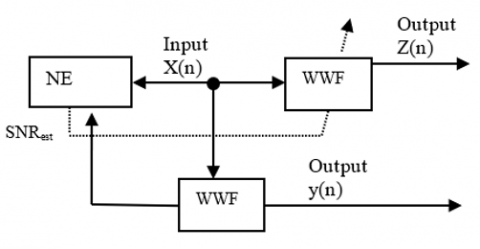Figure 3. Block diagram of the AWWF method

The model of noise would have a similar power spectrum as the muscle noise, where the model of the surface EMG signal is created by filtering a white Gaussian noise using a shaping filter H(f) which is given by Eq. (7) as follows:

$H(f)=\frac{f_{h}^{4} f^{2}}{\left(f^{2}+f_{l}^{2}\right)\left(f^{2}+f_{h}^{2}\right)^{2}}$    (7)

where, f h=120 Hz (high cutoff frequency) and fl =60 Hz (low cutoff frequency).

Right power of the noise is set to achieve required $S N R_{i n}$. It is done by multiplying the noise by a constant A. so that the input signal is given by Eq. (8) as follows:

x(n)=s(n)+Aw(n)    (8)

where, the constant A is given by Eq. (9) as follows:

$A=\sqrt{\frac{\sum_{n=0}^{N-1}[s(n)]^{2}}{10\left(\frac{S N R_{i n}}{10}\right) \sum_{n=0}^{N-1}[w(n)]^{2}}}$    (9)

2.3 Normalized least mean square (NLMS) adaptive filter algorithm

Adaptive algorithms are used to adjust the coefficients of the digital filter such that the error signal is minimized according to some criterion. In adaptive filters, the weight vectors are updated by an adaptive algorithm to minimize the cost function. A widely used linear adaptive filtering algorithm is named least mean square (LMS) algorithm by its originators, Widrow and Hoff (1960), it consists of two basic processes; filtering and adaptive process. LMS is simple to implement and gives a robust performance. However, it suffers from relatively slow convergence .

Figure 4 shows the structure of the based adaptive filter with an input sequence z(n) (from AWWF method) and with weights updated according to Eq. (10) as follows:

$f(n+1)=f(n)+\mu(z(n) e(n))$    (10)

where, f(n) are the filter coefficients, e(n) the estimation error, which is the difference between the desired response s(n) and the output of the filter f (n).

μ is the adaptation step size that determines the amount of correction applied as the filter adapts from one iteration to the next. A small step size achieves the optimum value but results in slow convergence, on the other hand; the large value of step size achieves faster convergence but results in missing the actual target.

## 4.png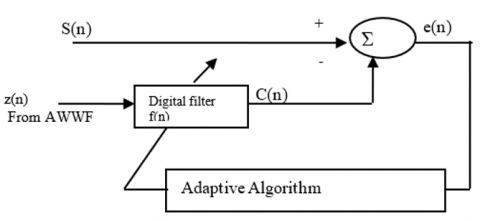Figure 4. Block diagram of Adaptive filter denoising

In this paper; the adaptive algorithm used for EMG noise reduction in ECG is Normalized Least Mean Square (NLMS) algorithm where the step size becomes adaptive. NLMS is another class of LMS algorithms it is different from LMS in its weight updating rule where convergence speed of the LMS filter depends on the input signal power (uses an adaptive step size based on the signal power). To make it independent, signal normalization is introduced. The expression of the Normalized LMS (NLMS) algorithm is given by Eq. (11) as follows:

$f(n+1)=f(n)+\frac{\mu}{z^{T}(n) z(n)}(z(n) e(n))$    (11)

where, $z^{T}(n) z(n)$ is the normalized signal input.

The block diagram of proposed combination (AWW and NLMS filtering) needs two inputs: S(n) the free original ECG signal, and Z(n) the noisy ECG signal obtained from AWW filtering.

The noisy ECG signal passes through the adaptive filter NLMS algorithm.

Thus, the adaptation of the filter coefficients and readjust itself continuously to minimize the error is fulfilled by the NLMS algorithm. And therefore, the output of the filter C (n) is a good estimate of S(n).

3. Results

The implementation and performance evaluation are done using MATLAB R2016b. The ECG signals used for testing are from MIT/BIH Arrhythmia Database where the recordings were digitized at 360 samples _per second. Forty-eight recordings were used for evaluation in this paper; they were pre-filtered using an averaging filter. The artificial noise used was generated using WGN function then it is shaped to EMG spectrum using a shaping filter. SNR in   is set from -5 to 30 dB in steps of 5 dB.

The results section consists of two parts named subsection 1, and subsection 2.

In subsection1:

• Five outputs of the proposed denoising combination are compared using five different thresholding techniques (Hard, Garrote, Hyperbolic, Semi Soft and Soft thresholding).
• The output of the proposed denoising combination is compared with the output of other techniques for denoising ECG signal such adaptive filtering , Butterworth low pass filtering , adaptive wavelet wiener with post LMS filtering  and adaptive wavelet wiener filtering .
• The output of the proposed denoising combination is compared with the original ECG signal.

In subsection 2:

• Achieved SNR of output signal and the Mean Square Error (MSE) of the proposed denoising combination is compared with achieved SNR of output signal and the Mean Square Error (MSE) of other techniques for denoising of ECG signal such as adaptive filtering, Butterworth low pass filtering , adaptive wavelet wiener with post LMS filtering and adaptive wavelet wiener filtering.
• Dependence of improvement SNR on input SNR after using AWWF and then after using the proposed denoising methodology is shown.

3.1 Subsection1 results

Figure 5 shows the comparison of five outputs of the proposed combination using five different thresholding techniques (Hard, Garrote, Hyperbolic, Semi Soft and Soft thresholding) for denoising noisy ECG signal from added white Gaussian noise to the data number of 105 from MIT/BIH Arrhythmia Database at 30 dB input, Figure 6 shows the comparison of different methods to denoise noisy ECG signal from added white Gaussian noise to the data number of 105 from MIT/BIH Arrhythmia Database at 30 dB input, Figure 7 shows the comparison between the reconstructed signal obtained by the proposed combination and the original ECG signal.

All the figures in subsection 1 (Figure 5, 6 and 7) display that the proposed combination (AWW and NLMS filtering) gives less distortion compared other ECG denoising methods, and display nearly similar shape as the original ECG signal.

## 5.png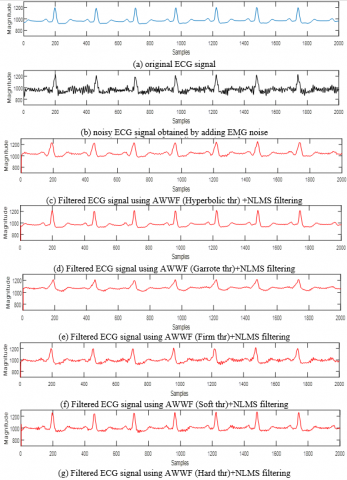Figure 5. Outputs of denoised ECG signal for input SNR of 30 dB and using LSMU threshold

## 6.pngFigure 6. Outputs of denoised ECG signal for input SNR of 30 dB using different denoising techniques

## 7.png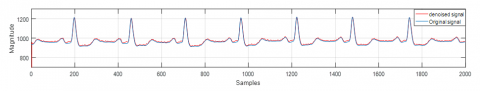Figure 7. Original and denoised ECG signal using adaptive wavelet wiener (with LSMU threshold) and NLMS filtering

3.2 Subsection 2 results

The performance of the different noise denoising techniques of the ECG signal is evaluated by calculating SNR output (SNRout), Mean Square Error (MSE) and SNR improvement (SNRimp) given by Eq. (12), Eq. (13) and Eq. (14) respectively as follows:

$S N R_{\text {out}}=10 \log _{10}\left(\frac{\sum_{n=0}^{N-1}[s(n)]^{2}}{\sum_{n=0}^{N-1}[C(n)-s(n)]^{2}}\right)[d B]$    (12)

$M S E=\frac{1}{N} \sum_{n=0}^{N-1}(s(n)-C(n))^{2}$    (13)

$S N R_{I m p}=S N R_{o u t}-S N R_{i n}$    (14)

Table 2 shows that the combination AWW+NLMS (using Garrote thresholding and LSMU threshold) gives better output SNR and lesser MSE compared to other methodologies like adaptive filtering, wavelet wiener filtering, Butterworth low pass filtering and AWWF+LMS filtering for denoising ECG signal of added white Gaussian noise at -5 dB to 30 dB input SNR to the ECG signals.

Table 2. SNRout and mean square error obtained after denoising ECG signal for SNRin=30dB

 Methods used SNRout (dB) MSE AWWF using LMSU threshold 43.256 0.00002522 AWWF using Tuning parameter TM  41.055 0.00004022 AWWF and NLMS filtering 52.816 0.00002304 AWWF and LMS filtering  48.389 0.00006993 Butterworth low pass filter  38.4849 0.00060880 Adaptive filtering  23.4495 0.00250200

Figure 8 shows Dependence of improvement SNR on input SNR, the average SNR improvement corresponding AWWF using LSMU threshold decreases from 24 to 6 dB with increasing input signal SNR while for proposed combination AWW+ NLMS filtering it decreases from 28 to 11 dB with increasing input signal SNR, where it is the better.

## 8.png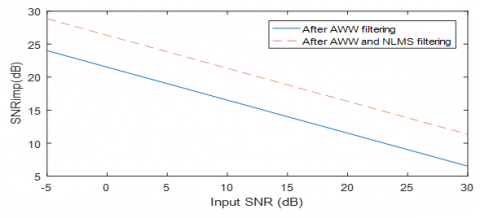Figure 8. Dependence of SNR imp on Input SNR

4. Discussion

In this paper, a new combination adaptive filter based on wavelet wiener transforms and normalized least mean square algorithm is introduced in the aim of denoising corrupted ECG signal from EMG noise. Both subsection results 1 and 2 show that the proposed adaptive filter achieved good results and outperforms other existing Denoising technique.

In subsection1 results, first the proposed combination using LSMU threshold and parameters introduced in Table 1 shows that: Hyperbolic with NLMS method (Figure 5c) results a widening in R wave and narrowing in Q wave, Firm with NLMS method (Figure 5e) results a widening in  R wave and distortion of the original ECG signal, using both of soft and hard with NLMS methods (Figure 5f, Figure 5g) the signal still keeps a lot of noise, but  using Non-negative garrote with NLMS method (Figure 5d) and sym8 for wavelet bank1, rbio1.1 for wavelet bank2 gets less distortion and nearly similar result as original ECG signal. Non-negative garrote has advantages over hard, soft, semi soft and hyperbolic thresholding rules in preserving the shape of QRS complex. The reason for these differences in the results obtained in this step is due to the successful combination between the smoothness of garrote rules, the accuracy of LSMU threshold from stage to stage, the optimal level that affects the shape of the signal and, the appropriate selection of the combination of filter banks (sym8, rbio1.1). Then in second comparison, AWWF using tuning parameter (Figure 6c) shows a widening in R wave due to the tuning parameter that prevents from correctly controlling the threshold when the signal changes suddenly, especially since it is constant in all levels. adaptive filtering method (Figure 6e) keeps QRS shape but a part in the beginning of the signal was omitted due to the step size parameter non adaptation characteristic of the least mean square algorithm. AWW with LMS method (Figure 6f) shows narrowing in Q wave due to the fixed threshold value in the first stage and to non adjusted step size in the second stage. Butterworth low pass filter method shows less noise but gives distortion of QRS complex (Figure 6g), while Butterworth is a not good choice for high frequency noise removal unless it is used in combination with another technique such wavelet transform. AWW with NLMS (Figure 6d) method using LSMU threshold shows best result compared to adaptive filtering, Butterworth low pass filtering, adaptive wavelet wiener with LMS filtering and adaptive wavelet wiener filtering for preserving QRS complex and removal added white Gaussian noise to the ECG signal.

The proposed combination of AWW with NLMS filtering also shows better SNR improvement and lesser MSE compared to other techniques as shown in subsection2 results. The reasons for better performance of our combination are appropriate selection of best wavelet filter banks, LSMU threshold value where it takes different thresholds at different scales, thresholding technique, adaptive step size and normalized signal input of the NLMS algorithm.

5. Conclusion

In this paper, an adaptive wavelet wiener based filter combined with Normalized Least Mean Square algorithm filter is proposed to remove EMG noise from real ECG signal. The Mean Square Error, achieved SNR and SNR improvement of the proposed method are calculated at different input SNR.

The use of SWT can increase the effectiveness of suppression of wide-band EMG noise in ECG signal by avoiding errors during reconstruction step of the decomposed signal and reducing the Pseudo-Gibbs phenomenon. The selection of the LSMU threshold value and garrote thresholding rule has an important influence on the preserving the shape of QRS complex of the signal and decrease the noise.

The NLMS-based adaptive filter is able to produce superior results in reducing EMG noise from the ECG signal obtained from wavelet-wiener based filtering compared to the conventional LMS adaptive filter exploiting the important characteristics which are: normalizing the power of the input and the adaptive step size. The proposed combination (AWWF with NLMS) shows lesser Mean Square Error, and better SNR improvement compared to other existing techniques.

References

 Camm, J.A., Lüscher, T.F., Maurer, G., Serruys, P.W. (2006). The ESC Textbook of Cardiovascular Medicine. Wiley-Blackwell.

 Sornmo, L., Laguna, P. (2005). Bioelectrical Signal Processing in Cardiac and Neurological Applications. Academic Press. https://doi.org/10.1016/B978-0-12-437552-9.X5000-4

 Paul, J.S., Reddy, M.R., Kumar, V.J. (1993). A transform domain SVD filter for suppression of muscle noise artefacts in exercise ECG's. IEEE Transactions on Biomedical Engineering, 47(5): 654-663. https://doi.org/10.1109/10.841337

 Thakor, N.V., Zhu, Y.S. (1991). Applications of adaptive filtering to ECG analysis: Noise cancellation and arrhythmia detection. IEEE Transaction Biomedical Engineering, 38(8): 785-794. https://doi.org/10.1109/10.83591

 Sharma, B., Suji, R.J. (2016). ECG denoising using weiner filter and adaptive least mean square algorithm. IEEE International Conference on Recent Trends in Electronics, Information & Communication Technology (RTEICT), Bangalore, India, pp. 53-57. https://doi.org/10.1109/rteict.2016.7807781

 Chawla, M.P.S. (2011). PCA and ICA processing methods for removal of artifacts and noise in electrocardiograms: A survey and comparison. Applied Soft Computing, 11(2): 2216-2226. https://doi.org/10.1016/j.asoc.2010.08.001

 El-Dahshan, E.A. (2011). Genetic algorithm and wavelet hybrid scheme for ECG signal denoising. Telecommuncation Systems, 46(3): 209-215. https://doi.org/10.1007/s11235-010-9286-2

 Donoho, D.L., Johnstone, I.M. (1994). Ideal spatial adaptation by wavelet shrinkage. Biometrika, 81(3): 425-55. https://doi.org/10.1093/biomet/81.3.425

 Li, S.Y., Lin, J. (2009). The optimal de-noising algorithm for ECG using stationary wavelet transforms. 2009 WRI World Congress on Computer Science and Information Engineering, Los Angeles, CA, PP. 469-473. https://doi.org/10.1109/csie.2009.999

 Kumar, S., Panigrahy, D., Sahu, P.K. (2018). Denoising of Electrocardiogram (ECG) signal by using empirical mode decomposition (EMD) with non-local mean (NLM) technique. Biocybernetics and Biomedical Engineering, 38(2): 297-312. https://doi.org/10.1016/j.bbe.2018.01.005

 Nikolaev, N., Gotchev, A. (1998). De-noising of ECG signals using wavelet shrinkage with time-frequency dependant threshold. 9th European Signal Processing Conference, Rhodes, pp. 2449-2453.

 Nikolaev, N., Gotchev, A. (2000). ECG signal denoising using wavelet domain Wiener filtering. Proc. European Signal Processing Conference, EUSIPCO, pp. 51-54.

 Nikolaev, N., Nikolov, Z., Gotchev, A., Egiazarian, K. (2000) Wavelet domain Wiener filtering for ECG denoising using improved signal estimate. 2000 IEEE International Conference on Acoustics, Speech, and Signal Processing. Proceedings (Cat. No.00CH37100), Istanbul, Turkey, 6: 3578-3581. https://doi.org/10.1109/icassp.2000.860175

 Chmelka, L., Kozumplik, J. (2005). Wavelet-based Wiener filter for electrocardiogram signal denoising. Computers in Cardiology, 32: 771-774. https://doi.org/10.1109/cic.2005.1588218

 Smital, L., Vítek, M., Kozumplík, J., Provazník, I. (2013). Adaptive wavelet wiener filtering of ECG signals. IEEE Transactions on Biomedical Engineering, 60(2): 437-445. https://doi.org/10.1109/tbme.2012.2228482

 El B’charri, O., Latif, R., Elmansouri, K., Abenaou, A., Jenkal, W. (2017). ECG signal performance denoising assessment based on threshold tuning of dual‑tree wavelet transform. BioMedical Engineering OnLine, 16: 26. https://doi.org/10.1186/s12938-017-0315-1

 Roopkanwal, Kaur, S.P. (2016). Electrocardiogram waveform denoising using a modified multi-resolution filtered-x LMS algorithm based on discrete wavelet transform. 2016 International Conference on Advances in Computing, Communications and Informatics (ICACCI), Jaipur, pp. 2162-2167. https://doi.org/10.1109/icacci.2016.7732372

 Coifman, R.R., Donoho, D.L. (1995). Translation-invariant de-noising. Wavelets and Statistics, 103: 125-150. https://doi.org/10.1007/978-1-4612-2544-7_9

 Pesquet, J.C., Krim, H., Carfantan, H. (1996). Time-Invariant orthonormal wavelet representations. IEEE Transactions on Signal Processing, 44(8): 1964-1970. https://doi.org/10.1109/78.533717

 Phinyomark, A., Limsakul, C., Phukpattaranont, P. (2009). EMG denoising estimation based on adaptive wavelet thresholding for multifunction myoelectric control. Innovative Technology Intelligent System and Industrial Applications, pp. 171-176. https://doi.org/10.1109/citisia.2009.5224220

 Liu, S. (2013). A novel thresholding method in removing noises of electrocardiogram based on wavelet transform. Journal of Information and Computational Science, 10(15): 5031-5041. https://doi.org/10.12733/jics20102845

 Ghael, S.P., Sayeed, A.M., Baraniuk, R.G. (1997). Improved wavelet denoising via empirical wiener filtering. Wavelet Applications in Signal and Image Processing V, 3169: 389-399. https://doi.org/10.1117/12.292799

 Rajmic, P. (2003). Exact risk analysis of wavelet spectrum thresholding rules. 10th IEEE International Conference on Electronics, Circuits and Systems, 2: 455-458. https://doi.org/10.1109/icecs.2003.1301820

 Simon, R. (1996). Adaptive filter theory. Prentice Hall; Upper Saddle River, NJ, USA. https://doi.org/10.1016/0967-0661(96)82838-3

 Salsekar, B., Wadhwani, A.K. (2012). Filtering of ECG signal using Butterworth filter and its feature extraction. International Journal of Engineering Science and Technology, 4(4): 1292-1298.

 Baby, N., Peter, S. (2015). Adaptive denoising of ECG signal using wavelet-based wiener filter and LMS filtering. International International Journal of Innovative Research in Computer and Communication Engineering, 3(11): 11689-11696. https://doi.org/10.15680/IJIRCCE.2015. 0311105